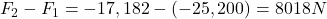## A family car has a mass of 1400 kg. In an accident it hits a wall and goes from a speed of 27 m/s to a standstill in 1.5 seconds. By how muc

Question

A family car has a mass of 1400 kg. In an accident it hits a wall and goes from a speed of 27 m/s to a standstill in 1.5 seconds. By how much would the force have been reduced if the car had had a crumple zone that increased the collision time to 2.2 seconds? Give your answer to the nearest whole number.

in progress 0
3 weeks 2021-08-26T18:31:08+00:00 1 Answers 0 views 0

The force has been reduced by 8018 N

Explanation:

The impulse exerted on the car during the crash is equal to the product of the force exerted and the duration of the collision, and it is also equal to the change in momentum of the car. So we can write: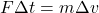where:

F is the force exerted on the car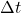is the duration of the collision

m = 1400 kg is the mass of the car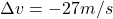is the change in velocity of the car

We can re-write the equation as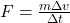In the 1st collision, the time is 1.5 seconds, so the force is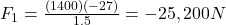In the 2nd collision, the time is increased to 2.2 seconds, so the force is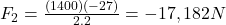Therefore, the force has been reduced by: